M362K Midterm Solutions, Given February 7, 2002

Problem 1. Light Bulbs

Suppose the probability function describing the life (in hours) of an ordinary 60 Watt bulb is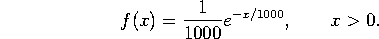Let A be the event that the bulb lasts 500 hours or more, and let B be the event that it lasts between 400 and 800 hours.

a) What is the probability of A?

A is the interval from 500 to infinity, so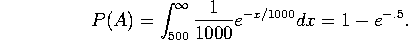(OOPS!  That should be e^(-1/2), not 1- e^(-1/2).  That's 5 points off for me.)

b) What is the probability of B?

B is the interval from 400 to 800, so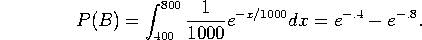c) What is P(A|B)?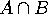is the interval from 500 to 800, so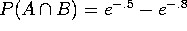, by a calculation almost identical to that above. Thus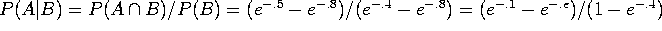.

Problem 2. Lawyers and Liars (20 points)

A fashionable club has 100 members, 30 of whom are lawyers. 25 of the members are liars, while 55 are neither lawyers nor liars.

a) How many of the lawyers are liars?

30 members are lawyers, so 70 are not.

25 members are liars, so 75 are not.

55 are neither lawyers nor liars, so there are 75-55=20 liars who aren't lawyers, and 70-55=15 lawyers who aren't liars.

That leaves 10 members who are both lawyers and liars. [You can also do this problem with a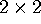table, or using the formula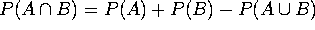.]

b) A liar is chosen at random. What is the probability that he is a lawyer?

There are 25 liars, of whom 10 are also lawyers, so the probability of a random liar being a lawyer is 10/25=40%.

Problem 3. Spring Break Sports

I haven't decided what to do over spring break. There is a 50% chance that I'll go skiing, a 30% chance that I'll go hiking, and a 20% chance that I'll stay home and play soccer. The (conditional) probability of my getting injured is 30% if I go skiing, 10% if I go hiking, and 20% if I play soccer.

a) What is the probability that I will get injured over spring break?

Let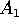be that I go skiing,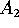that I go hiking, and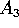that I play soccer. Let B be the event that I get injured. We are told that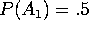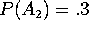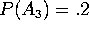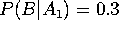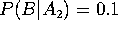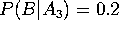. We can then calculate: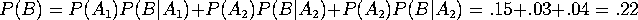b) If I come back from vacation with an injury, what is the probability that I got it skiing?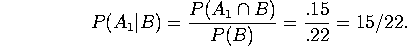Problem 4. Flipping Coins (30 points)

Suppose you flip a nickel, a dime and a quarter. Each coin is fair, and the flips of the different coins are independent.

Let A be the event ``the total value of the coins that came up heads is at least 15 cents''. Let B be the event ``the quarter came up heads''. Let C be the event ``the total value of the coins that came up heads is divisible by 10 cents''.

a) Write down the sample space, and list the events A, B, and C. Your answer should be of the form ``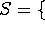(list of elements)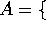(shorter list of elements)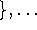''.

We'll order the coins nickel-dime-quarter, so ``HTT'' will mean that the nickel was heads and the dime and quarter were tails.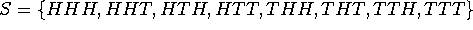. (Each of these 8 points has probability 1/8).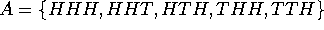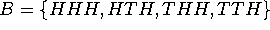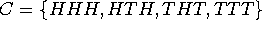b) Find P(A), P(B) and P(C).

Just count how many points are in each set to get P(A) = 5/8, P(B) = 1/2, P(C) = 1/2.

c) Compute P(B|A).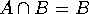, so P(B|A) = P(B)/P(A) = 4/5.

d) Are B and C independent? Explain.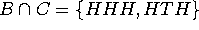, so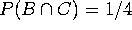. That is equal to P(B)P(C), so B and C are independent.